# Java Data Types

Challenge Inside! : Find out where you stand! Try quiz, solve problems & win rewards!
Video Tutorial## Overview

This article offers an introduction to Java data types, which are used to identify and categorize different data and determine the applicable operations. We will discuss two types of programming languages based on data types: statically typed (type decided during compilation) and dynamically typed (variable type can be changed).

We will also cover two main data type categories in Java: primitive (predefined) and non-primitive (programmer-defined). It provides insights into various primitive types like byte, short, int, long, float, double, char, and boolean. Additionally, we will discuss autoboxing, converting primitives to wrapper class objects.

## What are Data Types in Java?

Data Types mean to identify the type of data and associate operations that can be done on the data values. Data types define the value that a variable can take.

Data types also tell us information about:

• The size of the memory location.
• The maximum and minimum value of the range that can store in the memory location.
• Different types of operations can be done on the memory location.

## Types of Programming Languages

There are two types of programming languages based on the data types.

### 1. Statically Typed Language

Statically typed languages are those languages in which the type of data values or expression that variable will hold is decided during the compilation time of the program is called statically typed language.

The number variable only stores the integer value that is already decided during the compilation of the program.

JAVA language is a statically typed language, which means the type of particular variable is defined during the compilation time of the program that means in the above snippet the number variable is defined for storing int values if we try to assign other data values the java compiler will raise an error that is why java language is statically typed language.

### 2. Dynamically Typed Languages

As the name suggests, dynamic means that we can change the variable type after setting the particular type value to the particular variable. For example, the Python language is a dynamically typed language.

Python Code:

## Types of Data Types in Java

There are two types of data types in Java.

### 1. Primitive Data Types

Primitive data types are those data types that are predefined in the Java programming language.

There are a total of eight primitive data types that are predefined in the Java programming language. The size of the primitive data types does not change with changing the operating system because the Java programming language is independent of all the operating systems.

For example: byte, short, int, long, double, float, boolean, char

### 2. Non-Primitive Data Types

These are the data types that are not predefined but defined by the programmer according to the need for a particular task.

For example: String, Arrays, class, etc.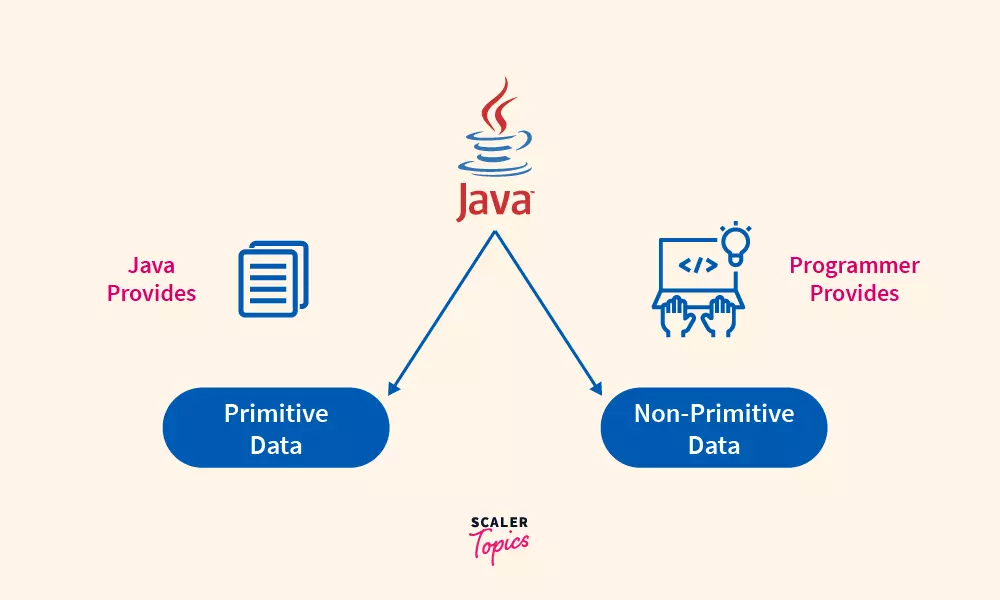Let’s move further to discuss the primitive data types but first think about why there are so many data types available in the Java programming language.

## Why There are So Many Data Types in Java?

Sometimes we have to deal with a large numerical data value, small numerical values, or decimal data values and we cannot use the same datatype again and again for storing the data values. For example, this is a bad practice to use long datatype for storing small-range values because it increases the memory usage of the program because the long data type uses more memory for storing the small-range values.

So to increase the efficiency of the program different kinds of data types are defined in the Java programming language although they are very similar in their functionality there is a huge difference in their properties (range, size allocation), etc. Let’s move further to understand these data types and their properties.

For a better understanding refer to the below chart

Classification chart of the Data types in Java: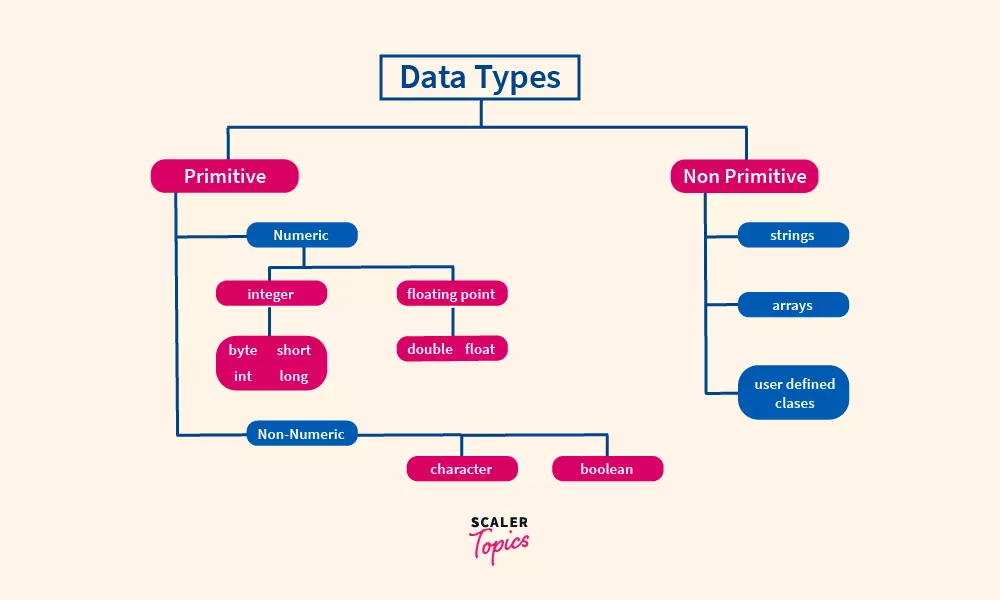## Types of Primitive Data Types in Java

Primitive data types are divided into two different types of values.

1. Numeric Data Types
2. Non-Numeric Data Types

## Numeric Data Types

Numeric data types are used to store numeric values such as whole numbers and fractional numbers. Numeric data types are divided into two parts, integer and floating.

### 1. Integer

Byte, short, long, and int these data types are used to store whole numbers. Let’s discuss these data types in detail.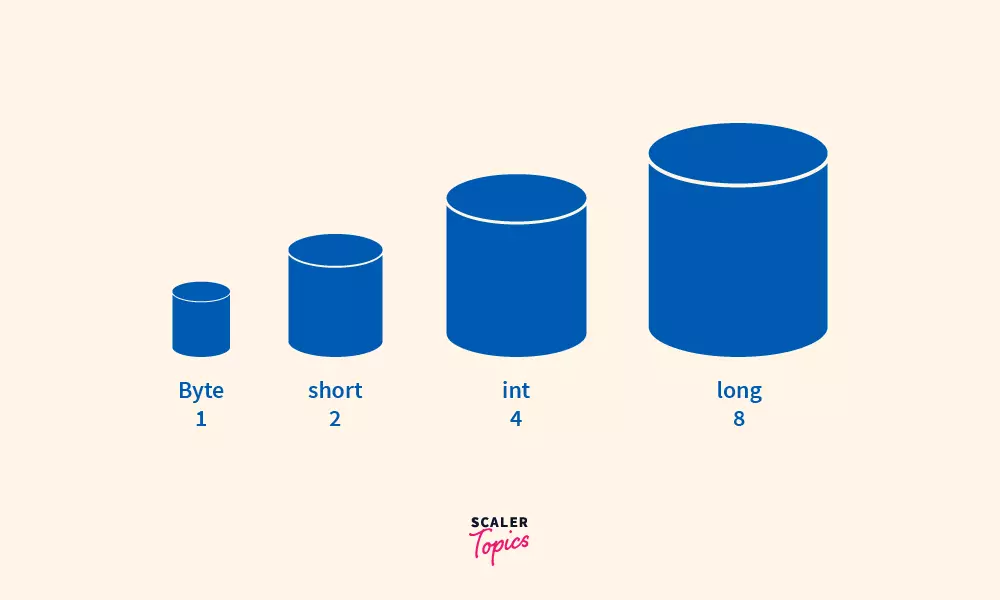A. Byte Datatype:

The byte data type is commonly used when we want to store very small numbers of very limited size. For example, the index record of the notebook.

Syntax:

• The byte data type can have data value in the range of -128 to 127.
• The byte data values require 1 bytes memory space in the computer’s memory.
• The default value of the byte is 0.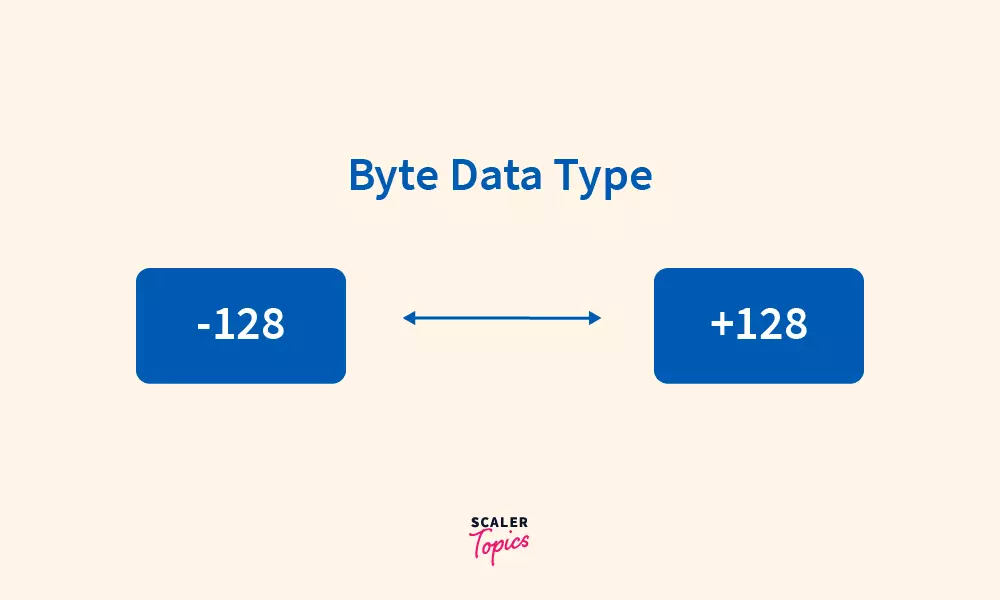Note: If the byte data type is initialized to the data value out of the above range, the compiler will show a type mismatch error due to the invalid date value initialized to the byte data type. Let’s understand this with the help of the below example.

Variable a is initialized to the valid range data value but variable b is initialized to the invalid data value and that’s why the compiler will show a mismatch error.

B. Short Data Type

The short data type is commonly used when we want to save memory in large arrays. For example, we can use a short data type for storing the admission number of the students of the school.

Syntax:

• The short data type can have data values- in the range of -32,768 to 32767.
• The short data values require 2 bytes memory space in the computer’s memory.
• The default value of the short data type is 0.

Note: If the short data type is initialized to the data value out of the above range, the compiler will show a type mismatch error same as in byte data type.

C. int Data Type

The int data type is commonly used when we want to save memory in large arrays. For example, we can use a short data type to store the admission number of the school’s students.

Syntax:

• The int data type can have data values in the range of -2,147,483,648 (-2^31) to 2,147,483,647 (2^31-1).
• The int data values require 4 bytes of memory space in the computer’s memory.
• The default value of the byte is 0.

D. Long Data Type

The long data type is used when the int data type cannot handle a wider range than the int data type range.

Syntax:

• The long data type can have data values in the range of -9,223,372,036,854,775,808 to 9,223,372,036,854,775,807.
• The long data values require 8 bytes of memory space in the computer’s memory.
• The default value of the long is 0.

In the above snippet, we are assigning a non-decimal number in the second variable of b which is out of the range of the long data type that causes an error

The above data types are used to handle non-decimal numbers.

Now, let’s discuss the data types that are used to store fractional numbers.

### 2. Floating Values Data Types

There are two types of Floating values data types in java that are used to store fractional number values.

E. Float Data Type:

Syntax:

• This data type is used to store numbers that have decimals up to 6 and 7 points. For example, 8.123456 this number can be stored in the float data type.
• The default value of the float data type is 0.0 and also we have to typecast the decimal values during initialization to float because by default the decimal is doubled in Java. So to prevent error we have to typecast the decimal values to float.
• The float data values require 4 bytes of memory space in the computer’s memory.

Example of Using Float Data Type:

In the above snippet, we are directly assigning the decimal values to the float data which causes an error because by default java considers decimal values as double data values and we have to typecast the decimal values to the float data values as we can see clearly in the second case.

F. Double Data Type:

The double data type is most commonly used for storing large decimal values for example the value of pi i.e. 3.1473…

Syntax:

• This data type is used to store numbers that have decimals up to 15 decimals
• The double data values require 8 bytes of memory space in the computer’s memory.

Example of Using Double Data Type in Java

In the above snippet, we discussed how to initialize the decimal values to the double data types.

## Non-Numeric Data Types

Two types of data types are used for storing non-numeric data types: char data type and boolean datatype.

G. Char Datatype

The char datatype is used to store a single character/letter enclosed in the single parenthesis.

Syntax:

• The character must be enclosed by single quotes.
• The default value of the char is ‘\u0000’.
• Memory allocation of the double data type is 2 bytes.
• The Range of char datatype is the ASCII value is -128 to 127.

Example of Using Char Datatype:

The above snippet tells us the valid use of the char datatype.

H. Boolean Datatype The boolean data type is used to store false or true boolean values.

Syntax:

• The boolean data type stores only two kinds of values i.e. false or true.
• The default value of the boolean data type is false.
• Memory allocation of the double data type is 1 byte.

Example of Using Boolean Datatype:

In the above program, the compiler will show a compiler error if any other kind of value other than true or false is assigned to the boolean data type.

We can clearly see that without creating the object of the primitive data types we are assigning the data values to the primitive data types that’s why Java is not a pure object-oriented language because it supports primitive data types like byte, short, int, long, etc that violate, the principal of the object-oriented programming language.

In the above, statement without creating the object of the int, we are assigning the data value to the variable and this is valid in the Java programming language. Although we can create the object of the primitive data types and they are termed as Wrapper class in java. The respective wrapper class of the primitive data types is given below.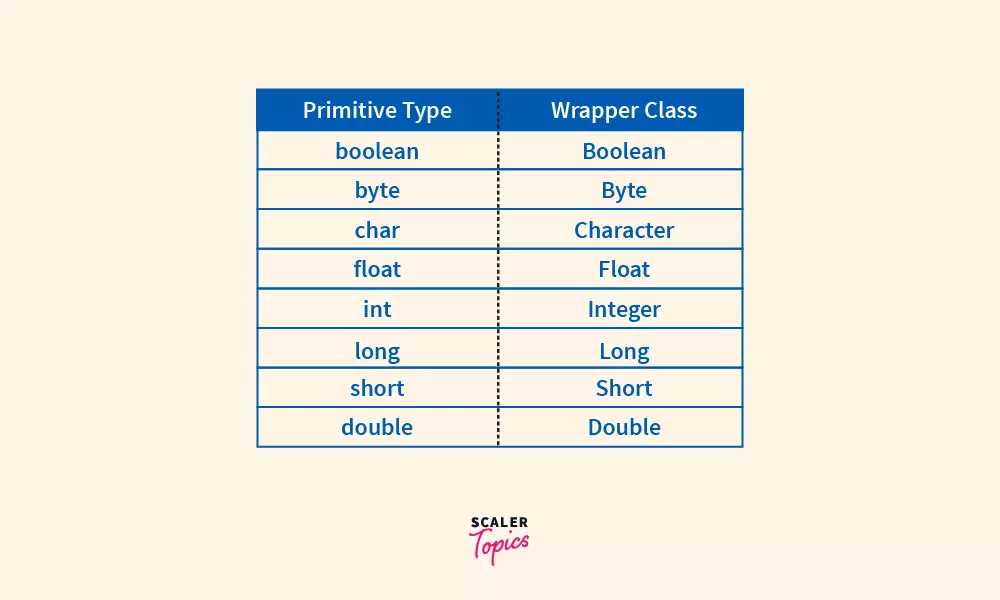## Conversion Primitive Data Types to Respective Wrapper Class

We can convert the primitive data types to their respective wrapper class objects and the process of converting the primitive data types to their corresponding wrapper class is called autoboxing.The main advantage of autoboxing is we can perform many operations on the data values that we cannot perform on the primitive datatypes because after autoboxing there are so many in-built functions that can be performed on the primitive types after performing autoboxing on the primitive data types. Let’s understand this concept with an example.

### Example

Line number 1 will show a compiler error because the primitive data type variable is not a reference of the object but after applying autoboxing the primitive type is converted to the corresponding wrapper class object and this object has multiple in-built methods that can be used for particular operations toString() is the function of the wrapper class. Use this Online Compiler to compile your Java code.

## Conclusion

In this article, We have discussed the primitive data types in Java and their features in the Java programming language.